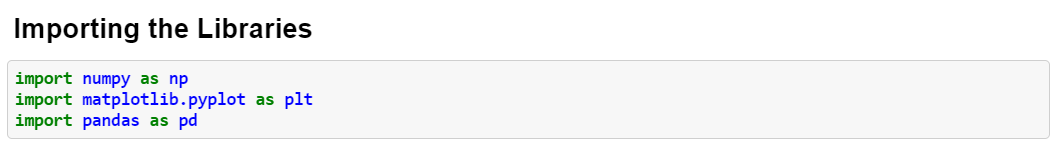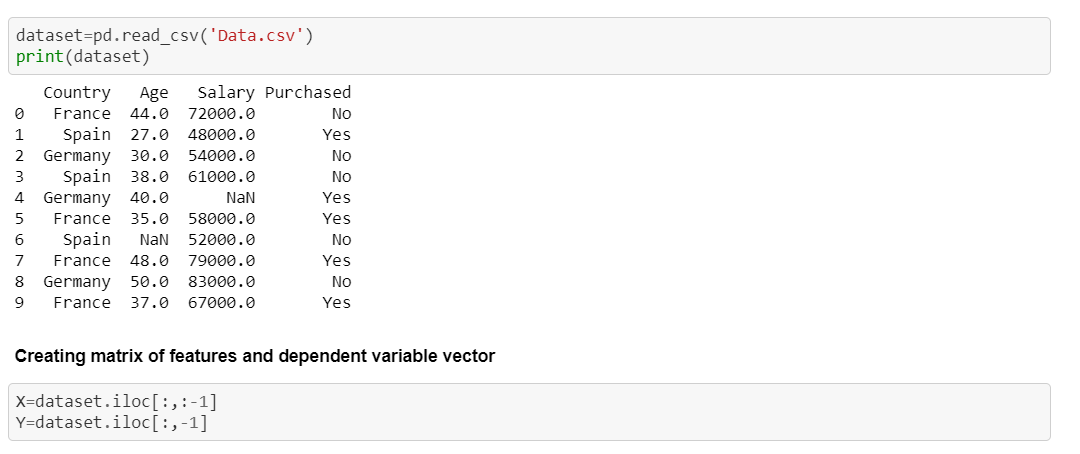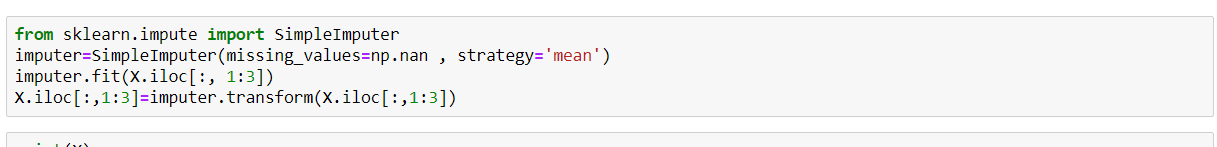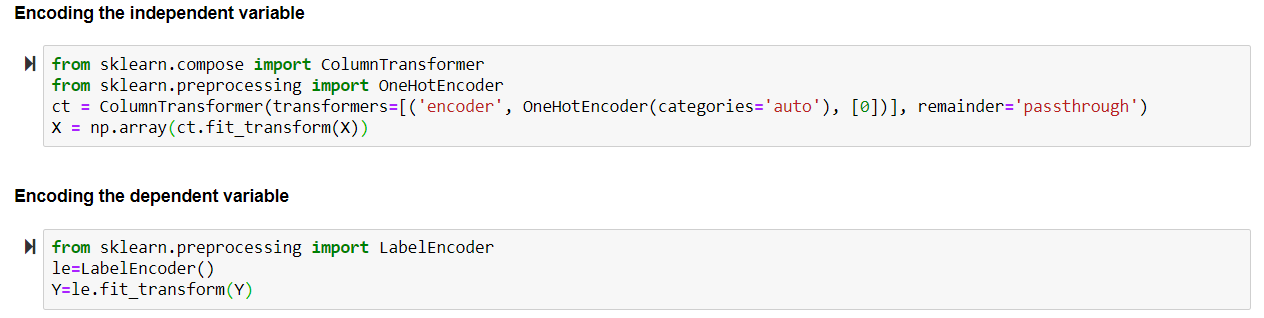Data preprocessing is an integral step in Machine Learning as the quality of data and the useful information that can be derived from it directly affects the ability of our model to learn; therefore, it is extremely important that we preprocess our data before feeding it into our model.

Every time you build a ML model you always have a preprocess phase to work on. So the ML model you are going to build can be trained in the right way on the data.

The concepts that I will cover in this article are-

1. Importing the Libraries
2. Importing the dataset
3. Handling the missing data
4. Encoding categorical data
5. Splitting the dataset into training set and test set
6. Feature Scaling

You can get the complete code (.ipynb) here.

Detailed analysis of steps is given below:-

# Importing the Libraries:

We have imported three libraries Numpy, Matplotlib, Pandas. A library is a symbol of modules containing functions and classes with which you can perform some actions and operations. Basically a library is super useful in building a machine learning model.

Numpy: It allows us to work with arrays.

Matplotlib: It allows us to plot some beautiful charts and graphs. We’ve used module pyplot for plotting charts.

Pandas: It allows us to import the dataset and also helps in creating matrix of features and the dependent variable vector.Python code for importing the libraries.

# Importing the dataset:

We have to create a new variable for storing our dataset. This function will read all the values of the dataset and will create a dataframe. We have to create two new entities:-

(i) Matrix of features/Independent Variable: These contain the information with which you can predict the dependent variable.

(ii) Dependent variable: These the values which you want to predict.Python code for importing the dataset.

## Why we have created these entities?

The way we’re going to build our future machine learning model it expects exactly these two entities in their input.

# Handling the missing data:

Missing data can cause errors in your machine learning model. Therefore you must handle it.

Method (i): Just ignore the observation by deleting it. This works only if you have a large dataset and 1% missing data. Removing 1% of data won’t affect the learning quality of the model. For lots of missing data, this is not the right approach.

Method(ii): Replace the missing value by the average of all the values in the column in which the data is missing. You have to change the index location according to your dataset.Python code for handling the missing data.

→fit method: For applying this imputer class to matrix of features, it will connect the features.

→transform: This will replace the missing values to the average of the values.

Important note: Apply missing value functions and methods only to numeric column data not to string and all others. You have to first convert them if you want to apply on them.

# Encoding Categorical data:

Idea 1: Encode every string to 1,2,3,…. likewise. But this is not fair as features like countries will be ranked, we can do this for features like sizes M,L,S,XS, etc.

Idea 2: One Hot Encoder

Turning one column into separate columns. It consists of creating binary vectors for each of the column.

(i) Encoding categorical independent variable.

Two classes used: (a) Column Transformer (b) One hot encoder

(ii) Encoding dependent variable.

Class used:

Label encoder: It will encode yes and no to 0 and 1.Python code to encoding the categorical data.

# Splitting the dataset into training set and test set:

Train set is where you’re going to train your machine learning model on existing observations. Test set is where you’re going to test the performance of your model on new observations.Python code for splitting the dataset into train and test set.

# Feature Scaling:

It puts all our features on the same scale. You don’t have to apply feature scaling to the dummy variables.

Two techniques:

(i) Standardization

(ii) Normalization

Class used : →Standard Scalar